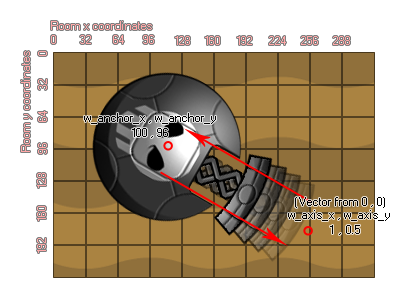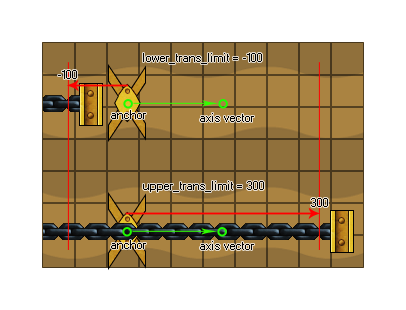# physics_joint_prismatic_create

## Create a prismatic joint connecting two instances and permitting movement along one fixed axis.

### 语法：

physics_joint_prismatic_create(inst1, inst2, w_anchor_x, w_anchor_y, w_axis_x, w_axis_x, lower_trans_limit, upper_trans_limit, limit, max_motor_force, motor_speed, motor, col)

inst1 The first instance to connect with the joint
inst2 The second instance to connect with the joint
w_anchor_x The x coordinate where the joint is anchored, within the game world
w_anchor_y The y coordinate where the joint is anchored, within the game world
w_axis_x The x component of the axis vector
w_axis_y The y component of the axis vector
lower_trans_limit The lower permitted limit for the joint movement
upper_trans_limit The lower permitted limit for the joint movement
limit Whether to limit the movement of the joint (true) or not (false)
max_motor_force Sets the maximum movement speed for the motor
motor_speed This is the speed at which the motor should move
motor Whether the motor should be active (true) or not (false)
col Whether the two instances can collide (true) or not (false)

Returns: index of the joint

### 描述

Like a revolute joint, the prismatic joint only has one degree of freedom, but with this joint it is directional relative to an axis rather than rotational and actually prevents any form of rotation. Here is an image to help you visualise how this works:We have two instances joined at the anchor point, with an axis for movement defined from the vector the two w_axis x/y coordinates relative to the (0,0) coordinates of the physics world (so an x component of 0 and a y component of 1 (0,1) would make the joint a vertical prismatic joint). This joint can then only move relative to this axis, like a spring or a piston. If you set the lower or upper trans limit, you are basically limiting the amount of movement along this axis, where the 0 position is the exact spot that you defined with w_anchor x/y, so a negative value would go to the "left" of that point along the axis and a positive value to the "right" as shown in the following diagram (realise that "left" and "right" are relative terms!):You can also define the joint as having a motor or not. This means that when un-influenced by a collision the joint will move in a direction along the axis. this direction is defined by the motor speed, with a positive number being towards the axis coordinates ("right") and a negative number being towards the anchor point ("left"). The "max_motor_force" argument is for limiting the speed of the movement so that you don't get a perpetually accelerating motor and to limit the influence that a collision may have on the movement. In this way you can use a joint motor to simulate joint friction by setting the joint speed to zero and maximum force to some small, but significant value. The motor will try to prevent the joint from moving, but will yield to a significant load.

As with all the joints, if you set the "col" value to true then the two instances can interact and collide with each other but only if they have collision events, however if it is set to false, they will not collide no matter what.

### 例如：

var mainFixture, o_id;
mainFixture = physics_fixture_create();
physics_fixture_set_circle_shape(mainFixture, sprite_get_width(sprite_index) / 2);
o_id = instance_create_layer(x+25, y, "Instances", obj_Piston);
physics_fixture_bind(mainFixture, id);
physics_fixture_bind(mainFixture, o_id);
physics_joint_prismatic_create(id, o_id, x, y, 0, 10, 0, 0, 0, 5, 0, 1, 1);
physics_fixture_delete(mainFixture);

The above code creates and defines a new fixture and then creates an instance of "obj_Piston", binding the created fixture to the two new objects. They are then joined by a prismatic joint with the anchor position at the same x/y coordinates of the first instance and an axis formed by the vector of the x/y position and the axis x/y (in this case 0,10, which is "down"). There are no limits placed on the amount of movement along this axis, but we have added a motor with 0 speed and a maximum force of 5 to simulate joint friction.